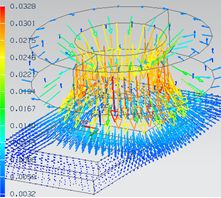# Physics

In this chapter the available physical solutions of the MAGNETICS for NX / Simcenter Solver are presented. Choose one solution to learn more about the theoretical background, the applications and the solved results of them.

# MagnetostaticsThe magnetostatic solution describes the distribution of static magnetic fields due to permanent magnets and continuous currents.

# ElectrostaticsThe electrostatic solution describes the distribution of electric fields due to static charges and / or levels of electric potential.

# Electrokinetics (DC)The electro-kinetic solution, also called 'DC Conduction Steady State', describes the distribution of static electric current in conductors.

# Coupled ThermalThe Coupled Thermal solution describes the distribution of temperature fields due to losses from electromagnetic solutions or due to applied thermal loads.

# Magnetodynamics - Time-domainThe magneto-dynamic solution describes the distribution of magnetic fields and eddy currents due to time dependent

# Electrodynamics - Time-domain

The electrodynamic solution describes the distribution of electric fields due to dynamically varying charges and/or levels of electric potential. This soluton allows time variations.

# High-Frequency - Time-domainThe Full Wave Solver takes into account all terms of Maxwell's equations. Due to this the wavelength can be smaller than the analyzed part dimensions and even very high frequencies are allowed. In the time domain results are the electric and magnetic field for every time step.

# Individual PhysicsThe powerful capability 'Individual Physics' allows the advanced user defining his own special physical formulations, solve for them in Magnetics and exploit all the excellent tools provided by NX / Simcenter.

# Magnetodynamics - Frequency-domainThe magneto-dynamic solution in frequency domain describes the distribution of magnetic fields and eddy currents due to time harmonic excitation loads.

# Electrodynamics - Frequency-domain

The electrodynamic solution describes the distribution of electric fields due to dynamically varying charges and/or levels of electric potential in frequency domain.

# High-Frequency - Frequency-domainThe Full Wave Solver takes into account all terms of Maxwell's equations. Due to this the wavelength can be smaller than the analyzed part dimensions and even very high frequencies are allowed. In the frequency domain Results are the electric and magnetic field due to excitation frequencies.

# Coupled ElasticityThe Coupled Elasticity solution computes mechanical displacements and stresses due to forces from electromagnetic solutions or due to applied mechanical loads.